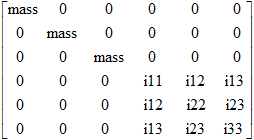# CONGM

Concentrated Mass ElementCONGM defines a concentrated mass element at an NLFE grid.

## Format

<CONGM
id       = "integer"
gid      = "integer"
mass     = "real"
[
x        = "real"
y        = "real"
z        = "real"
]
i11      = "real"
i22      = "real"
i33      = "real"
[
i21      = "real"
i31      = "real"
i32      = "real"
]/>

## Attributes

id
Unique identification number.
gid
Grid identification number.
mass
First grid identification number.
xyz
X, Y and Z offset distance from the grid point to the center of gravity. Default for x, y and z is 0.
i11
i22
i33
Mass product of inertia about X, Y and Z axes respectively.
i21
i32
i33
Mass product of inertia about X-Y, X-Z and X-Y respectively. Default value for all three attributes is 0.

## Example

The example demonstrates the definition of a CONGM element.

<CONGM id="1" gid="17" mass="10" i11="1" i22="1" i33="1"/>$\text{mass}=\int \rho dV$
$\begin{array}{l}i11=\rho \int \left({y}^{2}+{z}^{2}\right)dV=\rho \int {y}^{2}dV+\rho \int {z}^{2}dV\\ i22=\rho \int \left({x}^{2}+{z}^{2}\right)dV=\rho \int {x}^{2}dV+\rho \int {z}^{2}dV\\ i33=\rho \int \left({y}^{2}+{x}^{2}\right)dV=\rho \int {y}^{2}dV+\rho \int {x}^{2}dV\\ i12=-\rho \int xydV\\ i13=-\rho \int xzdV\\ i23=-\rho \int yzdV\end{array}$